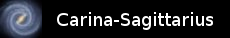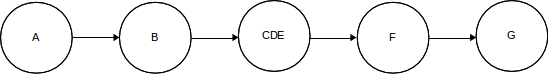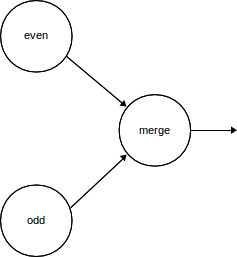# Programming Techniques

Programming a multi-processor that can execute a number tasks simultaneously requires different thought processes to those applied to conventional programming. For this multi-processor, it also requires a different way of looking at things compared to using multiple threads on a PC, because there is no shared memory. In many ways shared memory creates problems and has to be managed very carefully to avoid timing dependent behaviour. If independent tasks only exchange information by fully synchronised communication, some of the problems associated with shared memory are avoided, but there are still some pitfalls.

In purely sequential systems actions are performed one after another. Many programming languages use a semicolon to indicate this, i.e. “a1 ; a2” means perform action a1 before action a2. If actions a1 and a2 are composite, i.e. a1 is equivalent to “a11 ; a12 ; a13” and a2 is equivalent to “a21 ; a23”, the sequential composition of a1 and a2 is straightforward, i.e. “a1 ; a2” is equivalent to “a11 ; a12 ; a13 ; a21 ; a22”.

With parallel execution things are more complex. We might write “a1 || a2” to indicate that a1 and a2 are to be executed in parallel, but now there is no easy way to define how the execution of “a11 ; a12 ; a13” is sequenced with respect to “a21 ; a22”, i.e. what does “(a11 ; a12 ; a13) || (a21 ; a22)” mean? If any of the components of a1 access resources shared with the components of a2, there is no way to uniquely determining the end result without knowing the relative timing of all of the actions; something which may not be possible, or may vary from one execution to another. So even in a parallel execution environment, is important to be able to control the sequence in which actions are performed.

This multi-processor was designed to support systems which can be described by network diagrams such as that shown below in figure 4. It allows tasks to be executed simultaneously when a single processor would be forced to execute them sequentially, one after another. In sequential programs tasks that are independent often become tangled together simply because one processor has to execute them all. Therefore some simplification can also result from parallel execution. In many ways, the more computation a node can perform independently of every other node, i.e. without communication, the more effective the multi-processor will be.Figure 4

General networks like the one shown above can be built from components that fall somewhere between the 2 extremes shown in figure 5 and figure 6 below.

Figure 5 shows a disjoint parallel network where a number of nodes perform their tasks completely independently.Figure 5

Figure 6 shows a pipeline where nodes are connected together in a chain.Figure 6

In figure 5 the nodes C, D and E can perform their tasks completely independently of each other. In figure 6 the nodes A, B, CDE, F and G take it in turns to compute their results and pass them on to the next element in the chain. This does not imply that no simultaneous computation takes place. Initially, B may have to wait to be sent data by A before it can begin its computation, but A will be able to compute another result while B is working on the previous one. As results are propagated down the pipeline earlier nodes are free to compute further results.

The network shown in figure 5 can be substituted for node CDE in figure 6, and decisions can be made about how the outputs from B are connected to C, D, and E. Decisions can also be made about how the outputs from C, D and E are connected to F. The result is the network shown in figure 4. This demonstrates that figure 4 has the characteristics of both a pipeline and a disjoint parallel composition of nodes.

Non-determinism

Provided that nodes which receive data from many other nodes always use a separate port for each source, the behaviour of systems described in this way will be deterministic. That is, they will not depend on the rate at which nodes output results or the order in which the bus controller schedules communications.

Suppose that in the following system the node labelled even generates successive even numbered byte values less than 100 and the node labelled odd generates successive odd numbered byte values less than 100. Both send their values to the node labelled merge. the even node sends to port 1 and the odd node sends to port 2. It is then up to the merge node to decide which order to accept the outputs from even and odd. Suppose also that merge sends all the values it receives to its output. This simple network is shown in figure 7.Figure 7

Ignoring the details of a main program to configure this system and the full definitions of the classes defining the task of each node, the run functions for the even, odd and merge nodes might be defined as follows. For simplicity it is also assumed that the odd and even definitions have access to a constant that defines the node identifier of the merge node. In a full program this information would be provided by using links as demonstrated earlier in the sieve example.

//even

void run()
{
for (byte number = 0; number < 100; number = number + 2
{
send_to(number, merge_node_id, 1);
delay(interval_1);
}
}

//odd

void run()
{
for (byte number = 1; number < 100; number = number + 2
{
send_to(number, merge_node_id, 2);
delay(interval_2);
}
}

//merge

void run()
{
while (true)
{
send_to(even, output_connection);
send_to(odd, output_connection);
}
}

The 2 delays, using unspecified interval values, might represent the execution of some other calculations with unspecified execution times. If we run this system and observe the values that the merge node outputs, we will always find that it contains all the values from 0 to 99 in the correct sequence regardless of the relative length of the 2 delays, i.e. the system is completely deterministic.

Suppose instead that both even and odd send their values to the same port on the merge node. The 3 run functions would have to be rewritten as shown below.

//even

void run()
{
for (byte number = 0; number < 100; number = number + 2
{
send_to(number, merge_node_id, 1);
delay(interval_1);
}
}

//odd

void run()
{
for (byte number = 1; number < 100; number = number + 2)
{
send_to(number, merge_node_id, 1);
delay(interval_2);
}
}

//merge

void run()
{
while (true)
{
send_to(value, output_connection);
}
}

If we run this system, we would not be able to predict the order in which the merge node will output values without knowing the 2 delay intervals, which might be variable, and the precise speed at which computations are carried out, and the timing of communications across the bus. The behaviour of the system is severely non-deterministic.

It should be noted that the Sieve of Eratosthenes example described at the beginning of this article is potentially non-deterministic because all of the sieves send their primes to the printer node using the same port. The reason that the primes will be printed in ascending order is because each sieve sends its prime to the printer before it sends any values to the next sieve.

### Communicating Complex Data

The technique used to correctly sequence the odd and even numbers in the previous example can also be used in a more general way to make sure that, where several nodes wish to send complex data to another node, the receiver receives all the data from one sender before attempting to receive from another sender. The network involved might be organised as follows.Suppose that each sender is to send messages consisting of a single byte followed by two integers to the receiver. Each integer must be sent as 2 bytes because the bus can only handle one byte at a time. Therefore each message consists of 5 bytes in total. It is clearly important to make sure that the individual bytes sent by one sender do not get interleaved with the bytes from the other sender. If this were to happen, the receiver could input 2 bytes from different senders and combine them together to form an integer which neither sender had output. This can be avoided if the receiver receives the first byte on one port and then receives the two integers on another. Assuming for the purposes of the example that both senders are identical, the run functions for all 3, or in the general case more, nodes can be described as follows.

//sender_1 and sender_2

void run()
{
while (true)
{
byte byte_data;
int  int_data_1;
int  int_data_2;
/* compute data values here */
}
}

void run()
{
while (true)
{
/* process data here */
}
}

Each sender must wait until the receiver is prepared to receive a byte on port 1. It can then send a byte on port 1 and 2 integers on port 2. While this is happening, any further senders must wait until the receiver is ready to accept a byte on port 1 again. This guarantees that messages from different senders are not interleaved. It is clearly important that the senders respect the protocol implemented by the receiver. It is the programmer's responsibility to make sure that this is the case.

### Providing Shared Storage

Processing nodes cannot access each others local RAM, but a single node can make data stored in its RAM available to other nodes using a similar technique to that demonstrated in the previous example. In the following example, a single node will provide an array of values which can be updated by other nodes. After an update, the updated value is send back to the updating node. This might be used in a system which computes a histogram based on samples computed by a set of processing nodes.

//the store

void run()
{
const byte store_size = /* required store size goes here */;
byte data[store_size];
for (byte i = 0; i < store_size; i = i + 1) data[i] = 0;
while (true)
{
byte new_value          = data[element_to_update] + update_value;
data[element_to_update] = new_value;
send_to(new_value, user_node_id, 1);
}
}

//samplers

byte store_node_id = /* identity of store node goes here */

void run()
{
byte byte_data = /* initial data value goes here */;
while (true)
{
byte sample_value;
/* compute sample value */
byte sample_element;
/* choose an element to update */
send_to(this_node()     store_node_id);
send_to(sample_element, store_node_id);
send_to(sample_value,   store_node_id);
}
}

Each sampler causes the store to be updated by first sending its own node identifier to the store. The store then expects to receive an update value and the identity of the store element to be updated. It uses these to update the store and sends the updated value back to the sampler which started the update. While an update is in progress, any other sampler nodes wishing to update the store will have to wait until the store is ready to begin another update by accepting a new sampler node identifier.

### Acquiring and Releasing a Shared Resource

A single node can provide a shared resource similar to the store in the previous example, but such that once a client nodes has acquired access to it, the resource remains connected to the client while some variable number of transactions are performed. The client then releases the resource so that other nodes can used it. An example might be printing out a set of data via a serial line. It is clearly important that text sent by one node does not get interleaved with text sent by other nodes. The following functions could be used as part of the implementation of such a printer resource.

//printer resource

const byte control_port = 1;
const byte text_port    = 2;

void initialise()
{
Serial.begin(9600);
while (!Serial);
}

void run()
{
Serial.println("Printer Running");
while (true)
{
serve_client(client_id);
}
}

void serve_client(byte client_id)
{
Serial.print("Output by client ");
Serial.println(client_id);
Serial.println("-----------------------");
while (line_length >= 0)
{
while (line_length > 0)
{
Serial.print(ch);
line_length = line_length - 1;
}
Serial.println();
}
Serial.println("-----------------------");
}

The printer resource initialises the serial connection and prints a message to indicate that it is running. It then waits until it receives the node identifier of a client on port 1. It requires the client to send it, on port 2, a series of lines to print in the form of an integer line length followed by the corresponding number of bytes representing the characters to be printed for that line. A client can send any number of lines but must release the printer when it has finished by sending a negative line length. A blank line can be printed by sending a zero line length.

//printer client

const byte printer_control_port = 1;
const byte printer_text_port    = 2;

void run()
{
print_section_1();
print_section_2();
}

void print_section_1()
{
acquire_printer();
print_line("Debugging is twice as hard as writing the code in the first place.”);
print_line("Therefore, if you write the code as cleverly as possible,");
print_line("you are, by definition, not smart enough to debug it");
print_line("--Brian Kernighan");
release_printer();
}

void print_section_2()
{
acquire_printer();
print_line("Don't call it 'code' Brian! Coding implies encryption.");
print_line("'Source text' should be written as clearly as possible,");
print_line("and never encrypted.");
print_line("--Me");
release_printer();
}

void acquire_printer()
{
send_to(this_node(), printer_node_id, printer_control_port);
}

void print_line(String s)
{
send_int_to(s.length(), printer_node_id, 2);
for (int i = 0; i < s.length(); i = i + 1)
send_to((byte)s[i], printer_node_id, 2);
}

void release_printer()
{
send_int_to(-1, printer_node_id, printer_text_port);
}

Notice that the 2 sections of text generated by this client will always be printed in order with section 1 first. However, other clients may acquire the printer and produce output before, between, or after the 2 sections. A system with several printer clients, including the one shown above, might produce output like that shown below.

Printer Running
Output by client 5
-----------------------
Debugging is twice as hard as writing the code in the first place.
Therefore, if you write the code as cleverly as possible,
you are, by definition, not smart enough to debug it.
--Brian Kernighan
-----------------------
Output by client 3
-----------------------
The current temperature is 22C
-----------------------
Output by client 5
-----------------------
Don't call it 'code' Brian! Coding implies encryption.
'Source text' should be written as clearly as possible,
and never encrypted.
--Me
-----------------------
Output by client 7
-----------------------
The current relative humidity is 35%
-----------------------